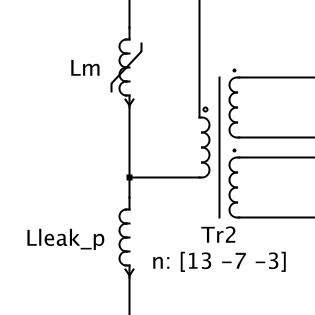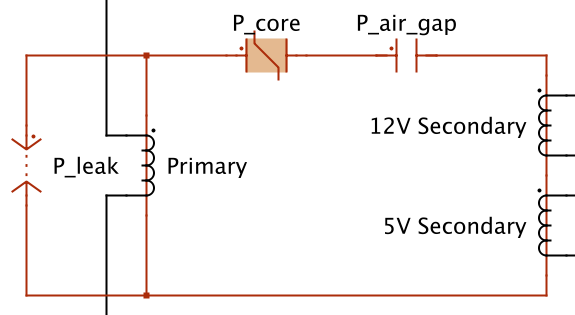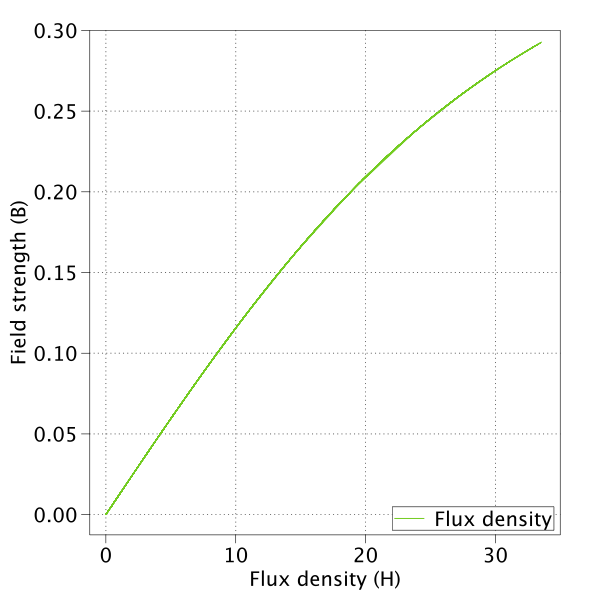# Flyback Converter with PLECS Magnetic Components

This PLECS demo model shows a DC-DC flyback converter operating in discontinuous conduction mode with two outputs. The converter has been designed for an average DC input voltage of 25 V. The two outputs are 12 VDC, 0.2 A peak and 5 VDC, 2 A peak, where the 12 VDC output is regulated with a control feedback loop. The model combines the electrical power circuit with a special magnetic circuit for the flyback transformer and the discrete controller.

### Power circuit with coupled inductor design for transformer

The power circuit is based on a standard flyback topology with two outputs. Rather than modeling the flyback transformer with an ideal transformer and magnetizing inductance in the electrical domain, primitive components from the PLECS Magnetic component library are used to model the saturable core, input and output windings, air gap, and leakage inductance.

Here are the electrical-equivalent and magnetic circuit designs for the transformer as implemented in PLECS:Electrical implementationMagnetic implementation

The transformer core was chosen to be a Ferroxcube 2616 pot-core made of 3C91 material. Using information from the datasheets, and the target output specifications, values were then calculated for saturation characteristics, the length of the air gap, leakage inductance, and the number of primary and secondary side windings. A plot of the saturation curve is shown below:An RCD snubber is used in the circuit to protect the switch during turn-off in from excessive voltage spikes. The snubber will dissipate the energy stored in the leakage inductance of the transformer and the capacitance on the switch (which has not been modeled in this example).

### Control

The controller is cascaded and consists of an outer voltage control loop, using digital PI control, and an inner current control loop operating in peak current mode. The voltage setpoint is 12 V for the high side output and the reference voltage for the PI controller is taken from this output.

### Simulation

The simulation shows a start-up of the power supply under rated load conditions for both outputs. At 0.1 s, the 12 V output has a load step to reach peak current conditions, and at 0.2 s the 5 V output has a load step to reach peak current conditions. A Scope displays the output voltages and currents and another shows the output power of each winding as well as the total. At start-up and after each load step the ramp-up of the voltage and currents to their target values is shown.

An XY Plot is also provided to show the BH loop for the saturation effect of the core. From the material datasheet, it is decided to not operate the core beyond 0.33 T and the XY plot shows that the core is driven close to this value without exceeding it.

### Try it

This model is available in the PLECS Demo Model library provided in both versions of PLECS.

Categories: